## Free Statistics

of Irreproducible Research!

Author's title
Author*The author of this computation has been verified*
R Software Modulerwasp_bootstrapplot1.wasp
Title produced by softwareBootstrap Plot - Central Tendency
Date of computationWed, 22 Dec 2010 17:51:05 +0000
Cite this page as followsStatistical Computations at FreeStatistics.org, Office for Research Development and Education, URL https://freestatistics.org/blog/index.php?v=date/2010/Dec/22/t1293040140cqttp5id2y3otzk.htm/, Retrieved Sat, 30 Sep 2023 13:16:30 +0000
Statistical Computations at FreeStatistics.org, Office for Research Development and Education, URL https://freestatistics.org/blog/index.php?pk=114440, Retrieved Sat, 30 Sep 2023 13:16:30 +0000
QR Codes:Original text written by user:
IsPrivate?No (this computation is public)
User-defined keywords
Estimated Impact114
Family? (F = Feedback message, R = changed R code, M = changed R Module, P = changed Parameters, D = changed Data)
-     [Univariate Data Series] [Arabica Price in ...] [2008-01-05 23:43:18] [74be16979710d4c4e7c6647856088456]
- RMPD  [Histogram] [histogram inflatie] [2010-12-22 13:21:55] [96348ef82925ade81ab3c243141d80f1]
-    D    [Histogram] [Historgram Inflatie] [2010-12-22 14:20:36] [30b3e197115d238a51c18bcedc33a6a5]
- RMPD      [Bootstrap Plot - Central Tendency] [blocked bootstrap] [2010-12-22 14:35:50] [30b3e197115d238a51c18bcedc33a6a5]
-    D          [Bootstrap Plot - Central Tendency] [notched boxplots ...] [2010-12-22 17:51:05] [03bcd8c83ef1a42b4029a16ba47a4880] [Current]
Feedback Forum

Post a new message
Dataseries X:
192,37
192,65
193,77
194,54
198,63
202,3
206,05
210,94
220,57
228,55
235,61
239,86
243,05
241,37
249,31
259,98
262,85
273,13
278,37
288,19
299,13
301,26
305,36
307,75
317,2
323,6
332,31
341,59
344,3
335,17


 Summary of computational transaction Raw Input view raw input (R code) Raw Output view raw output of R engine Computing time 4 seconds R Server 'RServer@AstonUniversity' @ vre.aston.ac.uk

\begin{tabular}{lllllllll}
\hline
Summary of computational transaction \tabularnewline
Raw Input & view raw input (R code)  \tabularnewline
Raw Output & view raw output of R engine  \tabularnewline
Computing time & 4 seconds \tabularnewline
R Server & 'RServer@AstonUniversity' @ vre.aston.ac.uk \tabularnewline
\hline
\end{tabular}
%Source: https://freestatistics.org/blog/index.php?pk=114440&T=0

[TABLE]
[ROW][C]Summary of computational transaction[/C][/ROW]
[ROW][C]Raw Input[/C][C]view raw input (R code) [/C][/ROW]
[ROW][C]Raw Output[/C][C]view raw output of R engine [/C][/ROW]
[ROW][C]Computing time[/C][C]4 seconds[/C][/ROW]
[ROW][C]R Server[/C][C]'RServer@AstonUniversity' @ vre.aston.ac.uk[/C][/ROW]
[/TABLE]
Source: https://freestatistics.org/blog/index.php?pk=114440&T=0

Globally Unique Identifier (entire table): ba.freestatistics.org/blog/index.php?pk=114440&T=0

As an alternative you can also use a QR Code:

The GUIDs for individual cells are displayed in the table below:

 Summary of computational transaction Raw Input view raw input (R code) Raw Output view raw output of R engine Computing time 4 seconds R Server 'RServer@AstonUniversity' @ vre.aston.ac.uk

 Estimation Results of Bootstrap statistic Q1 Estimate Q3 S.D. IQR mean 255.101333333333 260.658666666667 266.83 9.63566524635776 11.7286666666666 median 243.05 254.645 267.99 16.86277304835 24.94 midrange 266.98 268.335 268.335 2.3039851310432 1.35500000000002

\begin{tabular}{lllllllll}
\hline
Estimation Results of Bootstrap \tabularnewline
statistic & Q1 & Estimate & Q3 & S.D. & IQR \tabularnewline
mean & 255.101333333333 & 260.658666666667 & 266.83 & 9.63566524635776 & 11.7286666666666 \tabularnewline
median & 243.05 & 254.645 & 267.99 & 16.86277304835 & 24.94 \tabularnewline
midrange & 266.98 & 268.335 & 268.335 & 2.3039851310432 & 1.35500000000002 \tabularnewline
\hline
\end{tabular}
%Source: https://freestatistics.org/blog/index.php?pk=114440&T=1

[TABLE]
[ROW][C]Estimation Results of Bootstrap[/C][/ROW]
[ROW][C]statistic[/C][C]Q1[/C][C]Estimate[/C][C]Q3[/C][C]S.D.[/C][C]IQR[/C][/ROW]
[ROW][C]mean[/C][C]255.101333333333[/C][C]260.658666666667[/C][C]266.83[/C][C]9.63566524635776[/C][C]11.7286666666666[/C][/ROW]
[ROW][C]median[/C][C]243.05[/C][C]254.645[/C][C]267.99[/C][C]16.86277304835[/C][C]24.94[/C][/ROW]
[ROW][C]midrange[/C][C]266.98[/C][C]268.335[/C][C]268.335[/C][C]2.3039851310432[/C][C]1.35500000000002[/C][/ROW]
[/TABLE]
Source: https://freestatistics.org/blog/index.php?pk=114440&T=1

Globally Unique Identifier (entire table): ba.freestatistics.org/blog/index.php?pk=114440&T=1

As an alternative you can also use a QR Code:

The GUIDs for individual cells are displayed in the table below:

 Estimation Results of Bootstrap statistic Q1 Estimate Q3 S.D. IQR mean 255.101333333333 260.658666666667 266.83 9.63566524635776 11.7286666666666 median 243.05 254.645 267.99 16.86277304835 24.94 midrange 266.98 268.335 268.335 2.3039851310432 1.35500000000002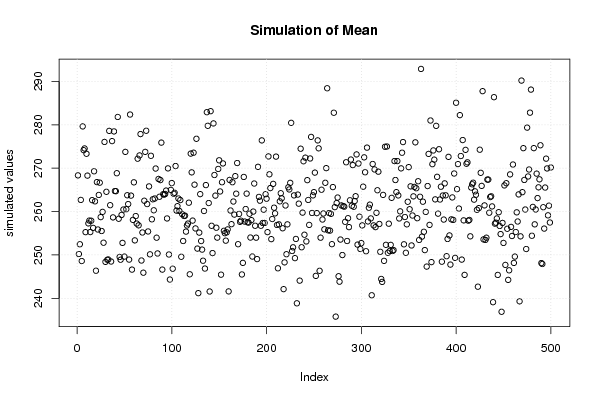PNG link Postscript link PDF link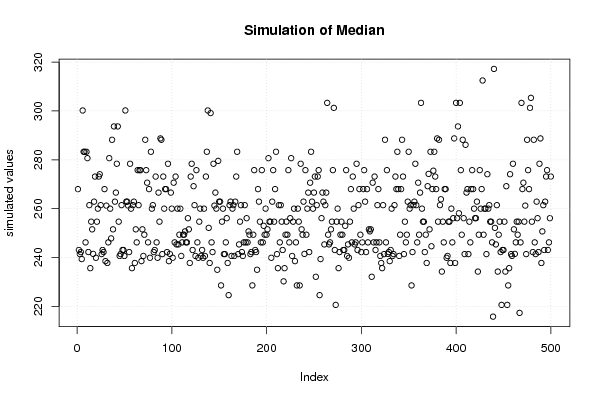PNG link Postscript link PDF link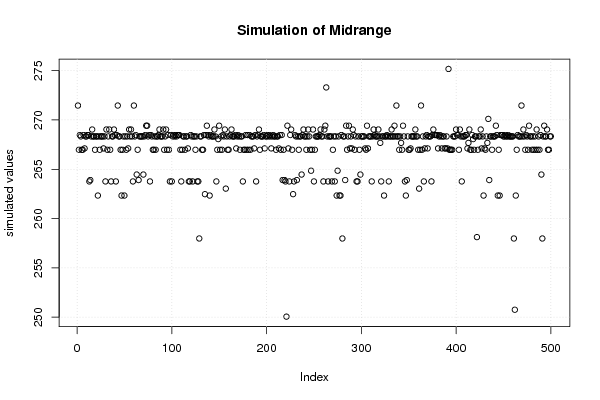PNG link Postscript link PDF link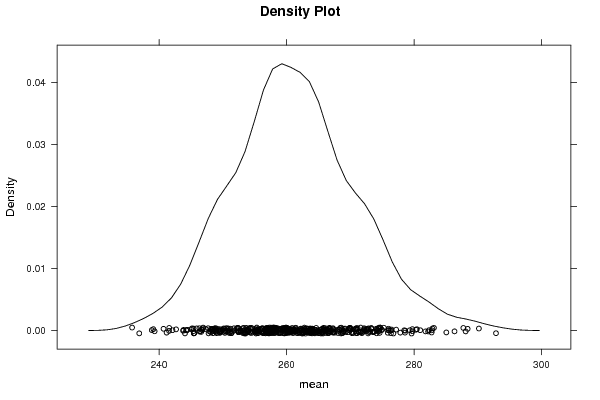PNG link Postscript link PDF link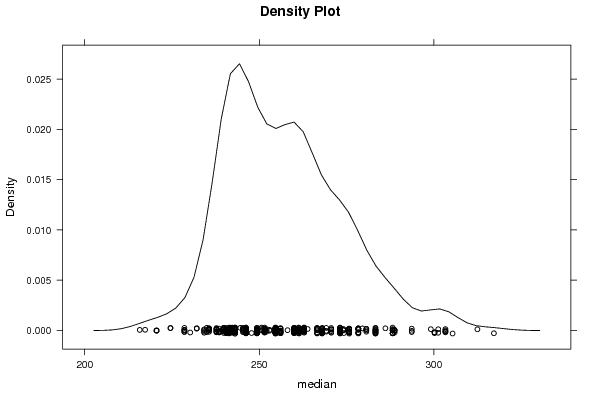PNG link Postscript link PDF link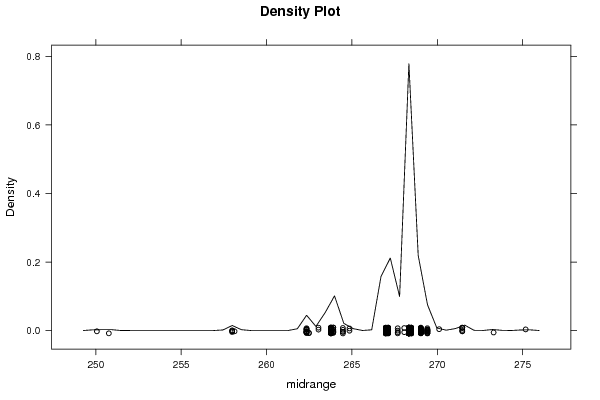PNG link Postscript link PDF link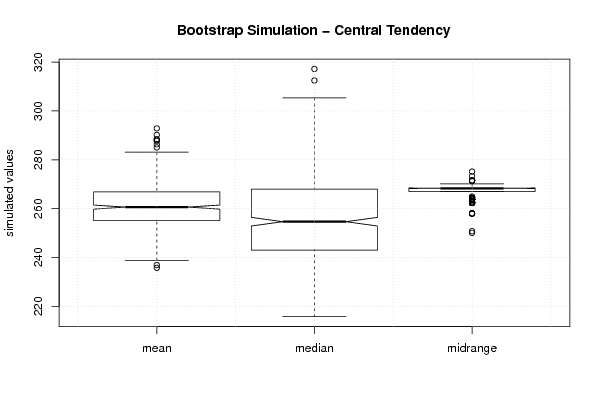PNG link Postscript link PDF link

Parameters (Session):
par1 = 500 ;
Parameters (R input):
par1 = 500 ;
R code (references can be found in the software module):
par1 <- as.numeric(par1)if (par1 < 10) par1 = 10if (par1 > 5000) par1 = 5000library(lattice)library(boot)boot.stat <- function(s,i){s.mean <- mean(s[i])s.median <- median(s[i])s.midrange <- (max(s[i]) + min(s[i])) / 2c(s.mean, s.median, s.midrange)}(r <- boot(x,boot.stat, R=par1, stype='i'))bitmap(file='plot1.png')plot(r$t[,1],type='p',ylab='simulated values',main='Simulation of Mean')grid()dev.off()bitmap(file='plot2.png')plot(r$t[,2],type='p',ylab='simulated values',main='Simulation of Median')grid()dev.off()bitmap(file='plot3.png')plot(r$t[,3],type='p',ylab='simulated values',main='Simulation of Midrange')grid()dev.off()bitmap(file='plot4.png')densityplot(~r$t[,1],col='black',main='Density Plot',xlab='mean')dev.off()bitmap(file='plot5.png')densityplot(~r$t[,2],col='black',main='Density Plot',xlab='median')dev.off()bitmap(file='plot6.png')densityplot(~r$t[,3],col='black',main='Density Plot',xlab='midrange')dev.off()z <- data.frame(cbind(r$t[,1],r$t[,2],r$t[,3]))colnames(z) <- list('mean','median','midrange')bitmap(file='plot7.png')boxplot(z,notch=TRUE,ylab='simulated values',main='Bootstrap Simulation - Central Tendency')grid()dev.off()load(file='createtable')a<-table.start()a<-table.row.start(a)a<-table.element(a,'Estimation Results of Bootstrap',6,TRUE)a<-table.row.end(a)a<-table.row.start(a)a<-table.element(a,'statistic',header=TRUE)a<-table.element(a,'Q1',header=TRUE)a<-table.element(a,'Estimate',header=TRUE)a<-table.element(a,'Q3',header=TRUE)a<-table.element(a,'S.D.',header=TRUE)a<-table.element(a,'IQR',header=TRUE)a<-table.row.end(a)a<-table.row.start(a)a<-table.element(a,'mean',header=TRUE)q1 <- quantile(r$t[,1],0.25)[]q3 <- quantile(r$t[,1],0.75)[]a<-table.element(a,q1)a<-table.element(a,r$t0)a<-table.element(a,q3)a<-table.element(a,sqrt(var(r$t[,1])))a<-table.element(a,q3-q1)a<-table.row.end(a)a<-table.row.start(a)a<-table.element(a,'median',header=TRUE)q1 <- quantile(r$t[,2],0.25)[]q3 <- quantile(r$t[,2],0.75)[]a<-table.element(a,q1)a<-table.element(a,r$t0)a<-table.element(a,q3)a<-table.element(a,sqrt(var(r$t[,2])))a<-table.element(a,q3-q1)a<-table.row.end(a)a<-table.row.start(a)a<-table.element(a,'midrange',header=TRUE)q1 <- quantile(r$t[,3],0.25)[]q3 <- quantile(r$t[,3],0.75)[]a<-table.element(a,q1)a<-table.element(a,r$t0)a<-table.element(a,q3)a<-table.element(a,sqrt(var(r\$t[,3])))a<-table.element(a,q3-q1)a<-table.row.end(a)a<-table.end(a)table.save(a,file='mytable.tab')